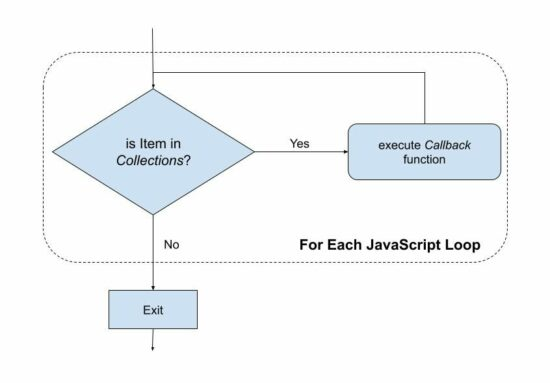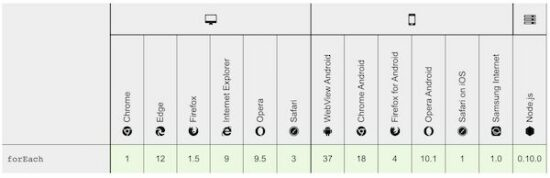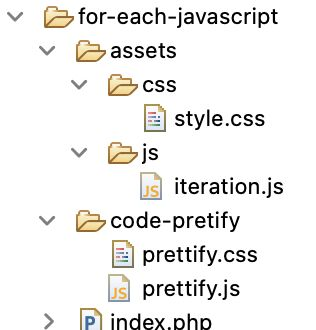# For Each JavaScript Array Iteration With Example

For Each in JavaScript is used for an array iteration. What’s new to learn about JavaScript For Each?  If you have this question, this article will definitely add value to your knowledge on this topic.

## Quick example

Iterates an array to print the array index and value.

``````function simpleIteration() {
var inputArray = ["Ant", "Bear", "Cat", "Dog", "Elephant"];
// For Each JavaScript array interation
inputArray.forEach((item, index) => {
document.write(index + ": " + item);
});
}``````

The For Each executes the callback function for every item in the iterating array in ascending order.

``````
forEach(function callbackFunction(item, [index], [arrayInstance]), [thisParameter])
``````

Continue reading to get in-depth knowledge on the JavaScript For Each. It covers syntax to shortcomings and alternative solutions.

Following are some of the interesting highlights that you will learn with JavaScript For Each loop.

• How to parse array and array-like objects.
• How to handle the array components index and items.
• How to handle array holes.

Almost all of the modern browsers are supporting For Each to loop through an array in JavaScript.

JavaScript has many alternatives for handling the array iteration. But, For Each serves in special-purpose situations. For example, you cannot skip or break the loop once started.## How to use JavaScript For Each

The JavaScript For Each function has two parameters.  Those are,

1. callback function.
2. this parameter (optional).

The callback function can have three parameters. And they are,

1. array-item
2. item-index
3. array-instance

The different usage syntax of the JavaScript for each is shown below.

### With external callback

The ‘this’ parameter is optional. It is used to pass a specific context to the callback to change the default. We will see an example to use forEach with this parameter.

The ‘this’ keyword refers to the object of the enclosing context. In a global scope, ‘this’ refers to the JavaScript window object. See how this works in JavaScript.

``````forEach(callbackFunction)
``````

(or)

``````forEach(callbackFunction, [thisParameter])
``````

### With inline callback

The callback function’s index and arrayInstance parameters are optional. The callback uses these parameters to perform array calculations.

``````forEach(function callbackFunction(item, [index], [arrayInstance]))
``````

(or)

``````forEach(function callbackFunction(item, [index], [arrayInstance]), [thisParameter])
``````

### With arrow function

The Javascript forEach can be used with the arrow function as below. The JavaScript arrow function is one of the features released by the ES6 (ECMAScript version 6).

``forEach(item => { // handle array })``

(or)

``````forEach((item, index, arrayInstance) => { // handle array })
``````

## Simple For Each JavaScript iteration

This code defines a JavaScript array of animals. It parses this array with For Each JavaScript iteration. It uses an inline callback function to prepare the output of the iterated array elements.

``````function simpleIteration() {
var result = "";
var inputArray = ["Ant", "Bear", "Cat", "Dog", "Elephant"];
// array iteration using For Each
inputArray.forEach(function(entry) {
result += "<div class='margin-bottom'>" + entry + "</div>";
});
document.getElementById("output1").innerHTML = result;
}``````

## For Each Example with this parameter

We have seen the optional ‘this’ parameter of the JavaScript forEach() function. Below example JavaScript code uses this parameter in the forEach callback.

In this example, the ‘this’ refers to the input array instance to append a new array of items into the existing array.

``````function appendNewItem() {
class AnimalList {
constructor(items) {
this.inputArray = ["Ant", "Bear", "Cat", "Dog", "Elephant"];
}

// For Each pushes new elements
array.forEach(function countEntry(entry) {
this.inputArray.push(entry)
}, this)
}
}

const obj = new AnimalList()

var result = "";
// For Each iterates and prepares output
obj.inputArray.forEach(function(entry) {
result += "<div class='margin-bottom'>" + entry + "</div>";
});
document.getElementById("output2").innerHTML = result;
}``````

## Handling the array instance, key, value in callback

In the above JavaScript forEach syntaxes, we saw how to handle the array parameters on the callback function.

The following JavaScript code defines forEach callback with item and index. The index param has the array key of the particular element in each iteration.

``````function handleArrayComponent() {
var result = "";
var inputArray = ["Ant", "Bear", "Cat", "Dog", "Elephant"];
// For Each iteration using index
inputArray.forEach(function(item, index) {
result += "<div class='margin-bottom'>" + index + " => "
+ item + "</div>";
});
document.getElementById("output3").innerHTML = result;
}``````

## JavaScript associative array iteration

The example code seen till now uses a simple input JavaScript array that has a default numeric index. Now, we are going to see how to iterate an associative array with the JavaScript forEach loop.

The below code uses JavaScript Map to create an associative array. We can also create an array object to associate the key-value together.

It prepares the output and appends it to the UI. It shows the key-value mapping of the input associative array.

``````function associativeArrayIteration() {
var inputArray = new Map([
['A', 'Ant'],
['B', 'Bear'],
['C', 'Cat'],
['E', 'Dog'],
['F', 'Elephant'],
]);

var result = "";
// For Each shows key value pair
inputArray.forEach(function(item, index) {
result += "<div class='margin-bottom'>" + index + " => "
+ item + "</div>";
});
document.getElementById("output4").innerHTML = result;

}``````

## JavaScript sparse array iteration

An array with uninitialised elements or holes is called a Sparse array. The JavaScript forEach leaves the array holes.

If you want to track uninitialised elements, choose an alternate instead of forEach.

The below JavaScript example supplies a sparse array to the forEach. It will print all the elements except the uninitialised.

``````function sparseArrayIteration() {
var inputArray = ["Ant", "Bear", , "Dog", "Elephant"];
var result = '';
// For Each skips array holes
inputArray.forEach(function(item){
result += "<div class='margin-bottom'>" + item + "</div>";
});

document.getElementById("output5").innerHTML = result;
}``````

## Array-like JSON object iteration

We have seen JSON parsing with PHP in an earlier tutorial. This code is about to iterate an array-like JSON object using the JavaScript forEach loop.

It accesses the JSON property with a reference of the property name in each iteration.

``````function jsonArrayIteration() {
var result = "";
var inputArray = [
{ "name": "Ant" },
{ "name": "Bear" },
{ "name": "Cat" },
{ "name": "Dog" },
{ "name": "Elephant" }
];
// For Each accesses JSON property
inputArray.forEach(function(item) {
result += "<div class='margin-bottom'>" + item.name + "</div>";
});
document.getElementById("output6").innerHTML = result;
}``````

## Iterating DOM collections in JavaScript

Iterating DOM collections in forEach processes the NodeList, HTMLCollections in a loop.

The below code calls .querySelectorAll to collect elements with a class selector reference. It returns a NodeList instead of an array.

By using getElementsByTagName()  or getElementsByClassName(), it returns HTMLCollection.

``````function domCollectionIteration() {
var inputArray = document.querySelectorAll('.dom-input');
var result = "";
// For Each prints HTML input value
inputArray.forEach(function(item) {
result += "<div class='margin-bottom'>" + item.value + "</div>";
});
document.getElementById("output7").innerHTML = result;
}``````

## Shortcomings of JavaScript For Each

The For Each Javascript method has some drawbacks and they are listed below.

• Skipping uninitialised elements of an array.
• Older browsers not supporting forEach and need an explicit prototype definition.
• Unable to break loop with the forEach() function.

## Polyfill for JavaScript array For Each

Some of the legacy browsers are not supporting the For Each JavaScript iteration method. To use For Each in such a situation, add the below code at the beginning of the script.

``````// Production steps of ECMA-262, Edition 5, 15.4.4.18
// Reference: https://es5.github.io/#x15.4.4.18

if (!Array.prototype['forEach']) {

Array.prototype.forEach = function(callback, thisArg) {

if (this == null) { throw new TypeError('Array.prototype.forEach called on null or undefined'); }

var T, k;
// 1. Let O be the result of calling toObject() passing the
// |this| value as the argument.
var O = Object(this);

// 2. Let lenValue be the result of calling the Get() internal
// method of O with the argument "length".
// 3. Let len be toUint32(lenValue).
var len = O.length >>> 0;

// 4. If isCallable(callback) is false, throw a TypeError exception.
// See: https://es5.github.com/#x9.11
if (typeof callback !== "function") { throw new TypeError(callback + ' is not a function'); }

// 5. If thisArg was supplied, let T be thisArg; else let
// T be undefined.
if (arguments.length > 1) { T = thisArg; }

// 6. Let k be 0
k = 0;

// 7. Repeat, while k < len
while (k < len) {

var kValue;

// a. Let Pk be ToString(k).
//    This is implicit for LHS operands of the in operator
// b. Let kPresent be the result of calling the HasProperty
//    internal method of O with argument Pk.
//    This step can be combined with c
// c. If kPresent is true, then
if (k in O) {

// i. Let kValue be the result of calling the Get internal
// method of O with argument Pk.
kValue = O[k];

// ii. Call the Call internal method of callback with T as
// the this value and argument list containing kValue, k, and O.
callback.call(T, kValue, k, O);
}
// d. Increase k by 1.
k++;
}
// 8. return undefined
};
}
``````

Note: To iterate the DOM collection by using the Polyfill definition use the below format.

``````Array.prototype.forEach.call(document.querySelectorAll(<selector-string>), function(item) {
// Handle array
});
``````

The MDN web documentation states the browser compatibility of the in-built array For Each Javascript iteration.## Alternatives of For Each JavaScript loop

The JavaScript provides several loop statements. Those are,

• for – basic way of parsing an array in JavaScript.
• while
• do … while
• for … in – loop through an array keys.
• for … of – loop through an array values.
• every() – checks every element passes the condition added in the callback.
• some() – checks at least one element passes the condition added in the callback.
• map()

The most frequently used loop statement is the for loop. It resolves the shortcomings of the forEach loop.

The below image shows the file structure of the JavaScript For Each example code. It has the JavaScript asset iteration.js to define forEach callbacks to parse types of array collections.

On a landing page, I show the code for each type of array iteration using forEach.## Conclusion

Hope this detailed article has helped you to learn the For Each JavaScript loop statement.

It pinpoints the how-to examples, drawbacks and alternatives to the forEach loop function.

## Comments to “For Each JavaScript Array Iteration With Example”

• redouane sehbani says:

hi Vincy thank you so much you re very helpful for humanity so thank you thank you thank you

• Vincy says:

Welcome Sehbani.

• Rob Salusbury says:

Hi Vincy,
Your tutorials and examples are absolutely awesome.
Thank you so much!
Best regards

• Vincy says:

Hi Rob,